Deutsche VersionHow does the sound or the noise depend on distance from the source?
● How does the sound decrease (fall-off, drop, or vary) with distance? ●
What is sound? What is
sound level? Sound, distance and 1/r law
How does sound propagate?
Damping of the sound with distance
Which effect has distance on the
propagation of sound out of doors?

 Sound waves are nothing more than pressure waves that enable the air and our eardrums to get in motion and let our eardrums and microphones vibrate. That is the sound we hear. Engineers should consider especially the sound pressure and its effect.; see: Sound pressure and Sound power  Effect and Cause Do not use the expression "intensity of sound pressure". Intensity is really not sound pressure. Compare: Sound pressure, sound pressure level, SPL, sound intensity, sound intensity level. How much is a twice (double, half) or three times louder sound? Sound? Which sound?   ● For calculations with sound levels (decibels) go to: "Damping of sound levels with distance".   How does distance affect sound? No frequency dependance. How do high frequencies affect sound? Frequency dependance.

Sound Pressure p and the Distance r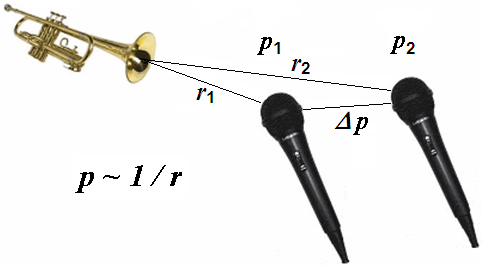There is no noise decrease or sound drop per meter. We get a sound level drop of 6 dB per doubling of distance. Sound power or sound power level has nothing to do with the distance from the sound source.

 Incidentally, the sound pressure p doesn't decrease with the square of the distance from the sound source (1/r²). This is an often-told and believed wrong tale.

 Sound power or sound power level has nothing to do with the distance from the sound source. Thinking helps: A 100 watt light bulb is emitting constantly the same power. That is really the case - no matter if in 1 m, in 10 m, or even in 100 distance. These emitted watts don't change with distance. They stay in the source. Sound power is the distance independent cause of this, whereas sound pressure is the distance-dependent effect.

 In a direct field or free field, the sound level (SPL) of a spherical wave decreases with doubling of the distance by (−)6 dB.   The sound pressure decreases in inverse proportion to the distance, that is, with 1/r from the measuring point to the sound source, so that doubling of the distance decreases the sound pressure to a half (!) of its initial value - not a quarter.   The sound intensity decreases inversely proportional to the squared distance, that is, with 1/r² from the measuring point to the sound source, so that doubling of the distance deceases the sound intensity to a quarter of its initial value.   Sound pressure (sound field quantity) is not sound intensity (sound energy quantity).   I ~ p2.   Loudnessis as a psychological correlate of physical strength (amplitude) is also affected by parameters other than sound pressure, including frequency, bandwidth and duration.

Sound means here "sound pressure deviations" as sound field quantity (1/r distance law).
Given: Reference distance r1, an other distance r2, and sound pressure p1 − Needed the other sound pressure p2.
 Reference distance r1  from sound source   m or ft Sound pressure p1 at reference distance r1  Pa = N/m² Another distance r2 from sound source   m or ft Sound pressure p2 at the other distance r2 Pa = N/m²

Sound Intensity I in the Distance rSound means here "sound intensity deviations" as sound energy quantity (1/ square law).
Given: Reference distance r1, an other distance r2, and sound intensity I1 − Needed the other sound intensity I2.
 Reference distance r1  from sound source   m or ft Sound intensity I1 at reference distance r1  W/m² Another distance r2 from sound source   m or ft Sound intensity I2 at the other distance r2 W/m²
 An "unclear" statement: The "sound" decreases with the square of the distance. Which sound exactly? Of course the sound that we hear as particle displacement ξ, sound pressure p, acoustic intensity I, acoustic power Pac, particle velocity v, sound energy density w. Is that really the case?

Sound pressure is not intensity

 Differentiate: Sound pressure p is a "sound field quantity" and sound intensity I is a "sound energyquantity". In teachings these terms are not often separated sharply enough and sometimes are even set equal. But I ~ p2. The sound power does not decrease with distance.

Changing of sound power with distance is nonsense

 Question: How does the sound power decrease with distance"? Answer: "April fool - The sound power does not decrease (drop) with distance from the sound source."   Levels of sound pressure and levels of sound intensity decrease equally with the distance from the sound source. Sound power or sound power level has nothing (!) to do with the distance from the sound source. Thinking helps: A 100 watt light bulb has in 1 m and in 10 m distance really always the same 100 watts, which is emitted from the lamp all the time. Watts don't change with distance.   A frequent question: "Does the sound power depend on distance?" The clear answer is: "No, not really."   We consider sound fields in air which are described by the scalar quantity p (sound pressure) and the vector quantity v (sound velocity) as a sound field quantity.

Sound pressure        Sound intensity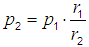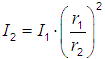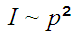Where: p1 = sound pressure 1 at reference distance r1 from the sound source p2 = sound pressure 2 at the other distance r2 from the sound source I1 = sound intensity 1 at reference distance r1 from the sound source I2 = sound intensity 2 at the other distance r2 from the sound source

Sound pressure formula    Sound intensity formula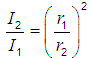Distance at sound pressure and distance at sound intensity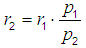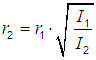Distance to the Sound Source
Distance-related decrease of sound

Sound means here sound pressure as sound field quantity.
Given: Sound pressure p1, p2, and reference distance r1 − Needed the other distance r2.
 Sound pressure p1 at reference distance r1  Pa = N/m² Reference distance   from sound source r1      m Sound pressure p2 at the other distance r2 Pa = N/m² The other distance   from sound source r2 m
Sound means here sound intensity as sound energy quantity.
Given: Sound intensity I1, I2, and reference distance r1 − Needed the other distance r2.
 Sound intensity I1 at reference distance r1  W/m² Reference distance   from sound source r1      m Sound intensity I2 at other distance r2 W/m² The other distance   from sound source r2 m
 The sound pressure decreases with 1/r at a distance from the sound source. The sound intensity drops with 1/r2 at a distance from the sound source. This is often confused and misunderstood because of the principal difference between the sound pressure as a sound field quantity and the sound intensity as a sound energy quantity is not known. The ear drums of our hearing and also the diaphragms of the microphones are moved effecttively by the sound pressure or the sound pressure level. Sound engineers should considerthis sound pressure as sound field quantity (size) more precisely; see: Sound pressure and Sound power − Effect and Cause

 Pressure as field quantity is never intensity as energy quantity.

 Formulas to calculate the sound pressure p or the sound intensity I in dependence of the distance r to a sound source.Decrease in level of sound pressure and sound intensity with distance

Inverse distance law 1/r for sound pressure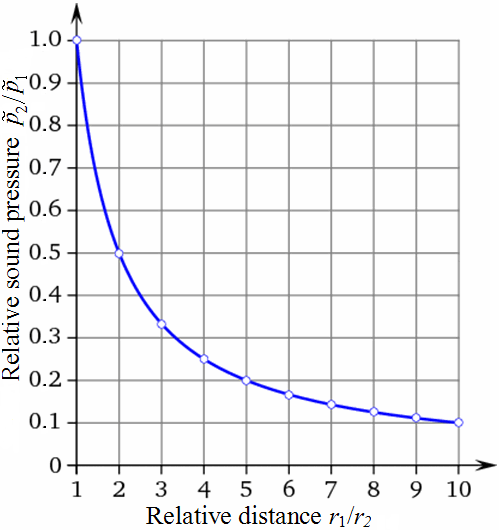Distance law for sound field quantities The graphs shown are normalized Distance ratio Sound pressure p1/r 1 1/1 = 1.0000 2 1/2 = 0.5000 3 1/3 = 0.3333 4 1/4 = 0.2500 5 1/5 = 0.2000 6 1/6 = 0.1667 7 1/7 = 0.1429 8 1/8 = 0.1250 9 1/9 = 0.1111 10 1/10 = 0.1000

Inverse square law 1/r2 for sound intensity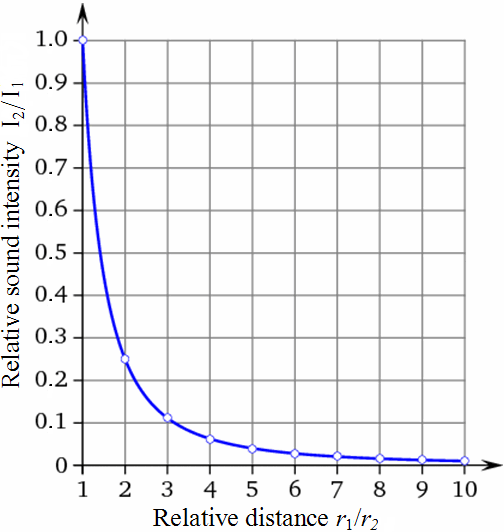Square law for sound energy sizes The graphs shown are normalized Distance ratio Sound intensity I1/r2 1 1/1² = 1/1 = 1.0000 2 1/2² = 1/4 = 0.2500 3 1/3² = 1/9 = 0.1111 4 1/4² = 1/16 = 0.0625 5 1/5² = 1/25 = 0.0400 6 1/6² = 1/36 = 0.0278 7 1/7² = 1/49 = 0.0204 8 1/8² = 1/64 = 0.0156 9 1/9² = 1/81 = 0.0123 10 1/10²=1/100 = 0.0100

Sound Level L and the Distance
Distance-related decrease of sound level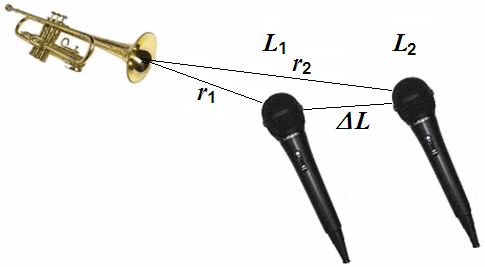Sound is here the sound level, whether it is the sound pressure level or the sound intensity level.
 Reference distance r1  from sound source   m or ft Sound level L1 at reference distance r1  dBSPL Another distance r2 from sound source   m or ft Sound level L2 at another distance r2 dBSPL
Calculation of the distance r2 where we get the specific sound level L2.
 Sound level L1 at reference distance r1  dBSPL Reference distance r1  from sound source   m Sound level L2 at another distance r2  dBSPL Another distance r2 from sound source   m
 Formulas to calculate the sound level L in dB (sound pressure level or sound intensity level) in dependence of the distance r from a sound source.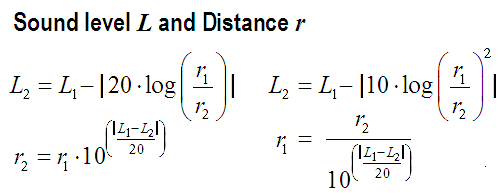Often we talk only of sound level. However, sound pressure as a sound field size is not the same as sound intensity as a sound energy size.   Levels of sound pressure and levels of sound intensity decrease equally with the distance from the sound source. Sound power or sound power level has nothing (!) to do with the distance from the sound source. Thinking helps: A 100 watt light bulb has in 1 m and in 10 m distance really always the same 100 watts, which is emitted from the lamp all the time. Watts don't change with distance.

What does sound level mean?

A reduction of the sound power level of the sound source by 6 dB is resulting in a reduction of the sound pressure level and the sound intensity level at the location of the receiver by also 6 dB, even if the sound power drops to a factor of 0.25, the sound pressure dropsto a factor of 0.5 and the sound intensity drops to a factor of 0.25. The reference value for the sound level was chosen so that with a characteristic acoustic impedance of Z0 = ρ · c = 400 N·s/m3 the sound intensity level results in the same value as the sound pressure level. We therefore simply speak of the "sound level" and leave it open whether sound pressure level or sound intensity level is meant.

 Sound engineers and sound protectors ("ear people") think by the short word "sound level" simply of "sound pressure level" (SPL) as sound field quantity.   Acousticians and sound protectors ("noise fighters") mean by the short word "sound level" probably "sound intensity level" as sound energy quantity. Equating sound pressure with sound intensity must cause problems.   I ~ p2.

Sound pressure level und sound intensity level

 To use the calculator, simply enter a value. The calculator works in both directions of the ↔ sign.
 Sound field size Sound pressure p:  Pa ↔ Sound level (SPL) Lp: dB 0 dB ≡ 0.00002 Pa and 1 Pa ≡ 94 dB
 Sound energy size Sound intensity I:  W/m2 ↔ Sound level LI: dB 0 dB ≡ 0. 000000000001 W/m² and 1 W/m² ≡ 120 dB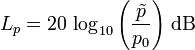Threshold of hearing = Reference sound pressure p0 = 20 μPa = 2 · 10−5 Pa ≡ 0 dB     Pa = N/m2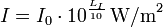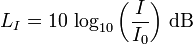Threshold of hearing = Reference sound intensity I0 = 1 pW/m2 = 10−12 W/m2 ≡ 0 dB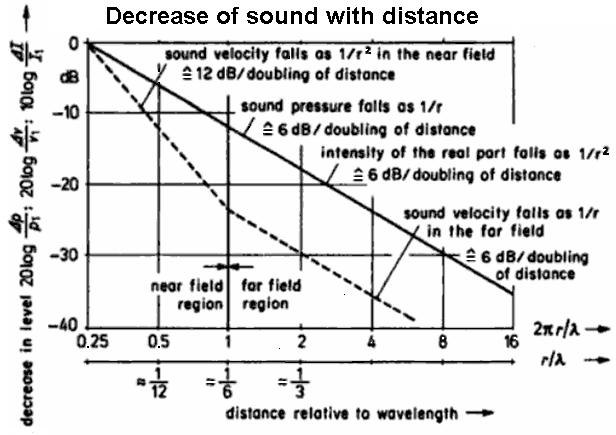Pressure, velocity, and intensity of the sound field near to and distant from a spherical radiator of the zeroth order

 For a spherical wave: The sound pressure level (SPL) decreases with doubling of the distance by (−)6 dB. The sound pressure falls 1/2 times (50%) of the sound pressure of the initial value. It drops with the ratio 1/r of the distance. The sound intensity level decreases with doubling of the distance by (−)6 dB. The intensity falls 1/4 times (25%) of the sound intensity of the initial value. Itdrops with the ratio 1/r2 of the distance.

 A spherical wavefrontis formed under the assumption of idealized conditions, such as a spherical radiator of zero order (ie, a "breathing" sphere) as a source for radiation in a homogeneous isotropic medium, usually air. For the dropping of sound pressure p and of particle velocity v we get in the far field: (r is the distance from the measurement point to the sound source).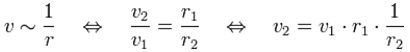All sound field sizes decrease in the far field after the 6-dB-distance law 1/r. Exception: The sound velocity goes with 1/r² in the near field . That is, the size values are halved by distance doubling. The sound intensity increases as the sound energy size is proportional to the square of the distance from the sound source decreases permanent from the sound source. Since the radiated sound power from the sound source as sound intensity is distributed on a growing area with the distance, the sound intensity falls off in the same proportion as the area grows larger.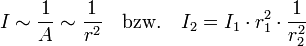The total radiated sound power remains stable in the theoretical model on an envelope to the spherical sound source, that is, power is independent of the distance r to the sound source.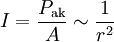Where: Sound power Pak in W, sound intensity I in W/m², distance from measuring point r in m, and area A in m².

 Sound engineers and sound designers ("ear people") are mainly interested in sound field sizes, and therefore consider the sound pressure drop at distance doubling. Acousticians and sound protectors ("noise fighters") are mainly interested in sound energy sizes, and therefore consider more the active intensity increase at distance doubling. All persons consider together the same line!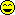Is this not beautiful? Nevertheless, the drop in sound pressure goes with 1/r and the decrease in sound intensity with 1/r2. This should be understood all right. If we are a sound engineer to review the sound quality by ear, then think of the soundwaves, which move the eardrums effectively by the sound pressure as sound field size. There is also the advice: Try to avoid to use the words sound power and sound intensity as sound energy sizes.

 We do not hear the air pressure changes as such, but the sound pressure at each ear, which is superimposed to the air pressure.

 In audio, electronics and acoustics use only the word "damping" and not the wrong word "dampening".   "damping" means: 1. a decreasing of the amplitude of an electrical or mechanical wave. 2. an energy-absorbing mechanism or resistance circuit causing this decrease. 3. a reduction in the amplitude of an oscillation or vibration as a result of energy being dissipated as heat.   "dampening" means: 1. To make damp. 2. To deaden, restrain, or depress. 3. To soundproof.   Notice: Damping is energy dissipation and dampening is making something wet.